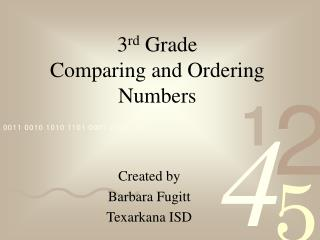# 3 rd Grade Comparing and Ordering Numbers - PowerPoint PPT PresentationDownload Presentation3 rd Grade Comparing and Ordering Numbers

3 rd Grade Comparing and Ordering NumbersDownload Presentation## 3 rd Grade Comparing and Ordering Numbers

- - - - - - - - - - - - - - - - - - - - - - - - - - - E N D - - - - - - - - - - - - - - - - - - - - - - - - - - -
##### Presentation Transcript

1. 3rd GradeComparing and Ordering Numbers Created by Barbara Fugitt Texarkana ISD

2. Vocabulary • Place value - The value of a place of a digit in a number. • Digits - Any one of the tens symbols 0, 1, 2, 3, 4, 5, 6, 7, 8, or 9 used to write numbers. • Greater Than (>) - A symbol used to compare two numbers, with the greater number given first. Example: 8 > 6 • Less Than (<) - A symbol used to compare two numbers, with the lesser number given first. Example: 6 < 8

3. Vocabulary • Equal (=) - Two numbers or number sentences being alike in value on either side. • Compare - To examine 2 or more objects or people to find out how they are alike and how they are different. • Ordering - To put numbers or objects in a specific order. Example: listing from greatest to least or least to greatest.

4. Comparing Numbers • Comparing numbers means to show one number is more than, less than, or equal to another number. • We use symbols to show the relationship. > = Greater than < = Less than = means equal to

5. Think of the signs like the mouth of an alligator. The alligator is greedy and always eats the larger number. 58 > 25 Greater than symbol

6. No matter which way he faces, he still wants to eat the bigger number. 25 < 58 Less than symbol

7. When two numbers are the same, you will use the equal sign to compare them. 25 = 25

8. Lets try some! 16 25 < 1,275 1,105 > 155 130 > 5,607 7,902 < 335 675 <

9. Ordering Numbers • Ordering numbers is putting numbers in a given order, either from least to greatest or greatest to least. • 1, 3, 7, 9, 11 is listed from least to greatest. • 11, 9, 7, 3, 1 is listed from greatest to least.

10. Ordering Numbers • Lets put these numbers in order from least to greatest. • To put these in order, start in the hundreds place. • Look at the 5, 3, and 7. Out of those three numbers, which is the smallest? 536 365 725 The 3 is the smallest number, therefore 365 is the smallest number.

11. Ordering Numbers • We see that 365 is the smallest number. • Now lets compare the 5 and the 7. • Which one of these two is smaller? 536 365 725 2 1 The 5 is the smallest number out of the two, therefore 536 is the next smallest number in the set.

12. Ordering Numbers • We see that 365 is the smallest number. • We see that 536 is the middle number. • Therefore, which is the largest number? • How would we list them? 536 365 725 2 1 3 The 725 is the largest number. We would list them as 365, 536, 725.

13. Lets try one.Put these numbers in order from least to greatest. 536, 126, 227 126, 227, 536

14. Lets try one.Put these numbers in order from greatest to least. 2,603; 5,892; 1,879 5,892; 2,603; 1,879

15. Which one belongs in the middle? • 136 C. 102 • B. 256 D. 302